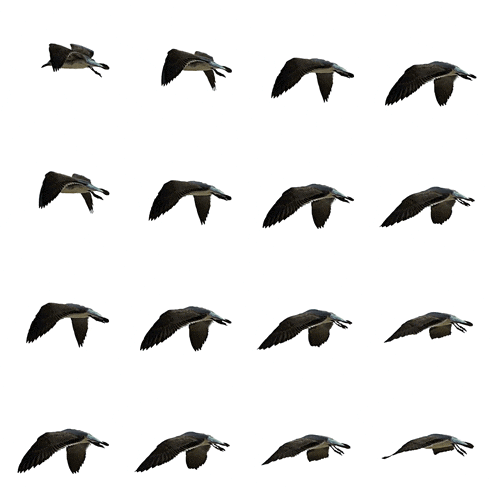Find Max. Paths to last Index in a 2D Matrix in JavascriptDhilip kumar twitter logo github logo Jun 6Updated on Jun 09, 2019・3 min readRead all my blogs here at My Website and follow me here on Twitter

It has been a while since my last blog.
I will be writing and explaining some algos as well as some interesting Javascript/React concepts that I come across.PROBLEM STATEMENT:

• Given the length of Row and Column of a 2D matrix.
• Starting from origin (0,0), find the maximum number of Paths one can take to reach the last index.
• Allowed ways to move => Right and Bottom.

Example:

• Let, row's length and column length be (2, 2)
• Starting from (0,0) one should reach (1,1)
• So the number of paths for that are: 2Path 1: (0,0) -> (0,1) -> (1,1)
Path 2: (0,0) -> (1,0) -> (1,1)

Thought Process:

Checking for a Pattern:

• We are allowed to traverse in two ways Down and Right for every Index. So this is a pattern.

Edge Cases:

• While we iterate we need to be careful about the ending index's count and so we should handle those cases.
• Path is 0 when the input is less than 1 for either row or column.(i.e.) Input cant be less than 1 for row length and column length

We found a pattern to go through for every index we can choose to solve with iteration / recursion.

Here we will solve it through RECURSION!const num_of_paths = findMaxPathSrcToDes(3, 3);
console.log('Number of Paths', num_of_paths);

We call findMaxPathSrcToDes and pass row length and column length and log it.

The intermediate Function:

function findMaxPathSrcToDes(rows, cols) {
// Initial rows and columns to begin with.0,0 is the first row and col index we are choosing
return findMaxPath(0, 0, rows - 1, cols - 1);
}
• findMaxPathSrcToDes function accepts the row length and column length from the user.
• It then returns the output from findMaxPath function to which we pass the origin which is (0,0) and destination index(rows -1, cols - 1).
• We can modify this origin and destination index to user defined positions by accepting those, so that we can identify the number of paths any index from any index.

Finding the Paths:

findMaxPath function takes in 4 params and outputs the number of path.

• currentRow - Which indicates the current index's Row that is being processed.
• currentColumn - Which indicates the current index's Column that is being processed.
• destRow - Destination row index
• destCol - Destination column index

In any Recursive solution, start with writing the base conditions or Exit conditions.

So, what is a Base condition or Exit condition?

It is basically the case on satisfying which our algorithm should terminate. So, let's formulate one.

• When currentRow > destRow (In this case it indicates that the currentRow count has gone out of bound).
• When currentColumn > destCol (In this case it indicates that the currentColumn count has gone out of bound). So we return '0' in both the cases.
function findMaxPath(currentRow, currentColumn, destRow, destCol) {
// Base condition
if (currentRow > destRow || currentColumn > destCol) {
return 0;
}
}

Success Case:

• if currentRow === destRow or currentColumn === destCol this indicates that we have reached the destination index so we return 1 to indicate a successful path.
if (currentRow === destRow && currentColumn === destCol) {
return 1;
}

Recursion Case:

• For each index there are two ways 1.Right and 2.Down
• So, we have to recurse in both ways and add the path formed from each ways.
• We call findMaxPath by incrementing currentRow by 1.
• Then again by incrementing currentColumn by 1 and adding the outputs of these two and return them.
const pathsInRows = findMaxPath(currentRow + 1, currentColumn, destRow, destCol);
const pathsInColums = findMaxPath(currentRow, currentColumn + 1, destRow, destCol);
return (pathsInRows + pathsInColums);

Next Step:

• You could try printing all possible paths along with count.Follow me for interesting contents.

That's All Folks!

DISCUSS

DEV is sort of like Medium, but it's open source and 100% focused on developers.

Now reaching over 3 million visitors per month, it's the fastest growing software development community in the world.

It's free, devoted to the open web, and will never have popups or a pay wall.

Classic DEV Post from Jul 10

How is your portfolio built?